Feeds:
Posts

## Modelling Distributed Generation using PowerWorld

My second “lab” for ECE4464: Power Systems II studied the effects of distributed generation (in particular, a large-scale wind turbine generation project) on the power system. Like ECE3333 (Power Systems I), this course is being taught by Prof. Rajiv Varma, Ph.D.

Using PowerWorld‘s Simulator software, we connected a large four-reactor nuclear generation plant (750MW per reactor, constant output) via two parallel transmission lines with a large city (modeled as an infinite bus). At the midline between the nuclear reactors and the large city, a transformer is installed at the midline bus to supply a different voltage to a nearby town. This town is near the proposed connection point of the Wind Turbine Generation (WTG) project; this project has a peak output of 85MW.

From the objectives:

In this lab, our objective is to study the potential impact of integration of distributed generation (in particular, a wind farm) using the PowerWorld Simulator software.  We simulate a very large capacity plant (a nuclear plant consisting of four reactors producing 750 MW each).  Because these reactors are large, they will provide some voltage regulation by supplying or consuming MVARs.

The nuclear plant serves a large city via two parallel transmission circuits, as well as the smaller city at the mid-line of one of these lines.  To simplify this lab, we model the large city as an infinite bus, which consumes any excess power generated by the plant.

In this manner, we can explore various phenomena resulting from distributed generation systems like wind farms, including the effects on power transfer and power system stability.  This lab provides insight on two very important issues in power systems, notably, the addition of distributed generation and the challenges involved with electrifying remote communities.

For my full report, see: Power Systems 4464 Lab 2 (PDF). Note that the small town bus has a constant 20Mvar reactive power demand, with a 40Mvar (nominal) shunt capacitor installed initially. Some modifications are made to the compensation scheme as part of the study and report.

## A Quick Primer on Power Factor

In the study of electrical engineering, power factor comes up quite often in terms of its various mathematical definitions, but people seem to overlook its real-world relevance. Though there are some regulations governing power factor, the way residential users are billed for electricity often leaves us in blissful ignorance of the importance of power factor. In fact, power factor is a measure of efficiency.

Starting from first principles, let’s look at the equation for instantaneous power in electrical systems: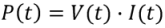Of course, there are similar definitions for mechanical power (which involve torque and speed rather than voltage and current as above).

In the best case, the voltage and current waveforms will be identical, which means that they are both sinusoidal with crests and troughs occurring together. For passive devices like light bulbs (which are purely resistive loads), this is exactly what happens. However, some devices (such as capacitors and inductors) store energy for a short period of time, causing the waveform of the current to be phase shifted or displaced, relative to the voltage wave.

If we use the “average” values of voltage and current, we can determine what is known as apparent power. Even though I call them average, what we really use are the “root-mean-square” values — the reasons we use this measure are beyond the scope of this article, but suffice it to say that we can’t use a normal average for sine since it would simply be zero. For you statisticians out there, RMS is related to standard deviation (it’s a special case where your mean is zero).Though its units are identical to those of Power (Watts), we use a different unit convention for this value, the Apparent Power (Volt-Amps, or VA).

Power factor is simply the ratio of real power compared to apparent power: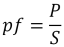For linear devices which do not store energy, real power and apparent power are the same, so the power factor is 1 (sometimes people call this “unity power factor”).

If, however, an energy storage device like an inductor or capacitor stores energy and simply return it back to the source, then the power factor will be reduced (since power is being transmitted over the line, stored temporarily and then sent back). Only real power contributes to work actually done — whether it be heating your room or turning a motor.

As mentioned earlier, inductors and capacitors cause the voltage and current to be shifted relative to each other. This results in what is called the Displacement Power Factor (the angle, φ, refers to the angular displacement between voltage and current):However, as we are moving forward in semiconductor techologies, we are increasingly encountering more and more nonlinear devices which introduce something known as Harmonic Distortion. Basically, it makes the current waveform “noisy” compared to the voltage reference; usually this is because a device switches on and off (goes from periods of drawing some current to zero current) instead of merely being proportional to applied voltage.

Total harmonic distortion (THD) is a measure of how “noisy” the current waveform is; for example, if you draw lots of power in short-duration bursts, the current wave won’t look like a sinusoidal waveform at all. This is also known as a shape factor since it will be 1 (unity) for perfectly sinusoidal current, and smaller otherwise.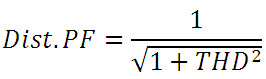THD is measured as a percentage, so its value is somewhere between 0 and 1.

The overall power factor takes both distortion (current waveform shape) and displacement (current waveform phase difference, relative to the voltage) into account:So now we have identified an equation useful for determining the overall power factor of your equipment, especially for things like computers, cooking, heating, washing/drying of clothes, etc. But what does power factor have to do with efficiency?

To answer that question, let’s rearrange our power factor vs (real and apparent) power equation to look at what happens to current. We’ll be looking at RMS current, which is important because it is one of the primary factors that determines maximum loading of a given power line.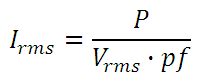Since the amount of power we’ll need and the RMS voltage (line voltage) are effectively fixed, we can see that power factor and RMS current are inversely related. That is, with a lower power factor, we will require higher RMS current to deliver the same amount of power to our load; our RMS current is lowest when the power factor is 1 (unity).

Since power dissipated across a transmission line is: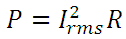There are finite limits on the amount of current that can be transmitted (greater power dissipated results in more heating of the wires, which can cause them to expand significantly or to melt), a unity power factor means a more effectively utilized infrastructure.

If everything we connect to the power system has a low power factor, it will result in an inefficient use of existing infrastructure (since we are transmitting more current than necessary, which also increases total line losses). It also means we will need a greater investment in infrastructure sooner, which is a challenging issue facing electric power utilities responsible for distribution of power.

## Power Transfer Simulation using PowerWorld

Recently, I completed my first “lab” for ECE4464: Power Systems II. Like ECE3333 (Power Systems I), this course is being taught by one of the most inspiring professors I have ever had, Prof. Rajiv Varma, Ph.D.

Using PowerWorld‘s Simulator software, we repeated one of our basic labs from ECE3333 as an introduction to computerized modelling of power systems. We connected a single synchronous machine to an infinite bus across a 600km, 1000MW-SIL power line.

It is simplest for me to just lift the objectives from my lab report:

In this lab, our objective is to simulate a simple single machine infinite-bus configuration using the PowerWorld Simulator software.  We design a local generator system (a synchronous generator) having a nominal generation capacity of 500MW and with no predefined peak generation (that is, the generator is modelled as having infinite generation capability).

In this manner, we can explore various phenomena like power transfer, power system stability and the effect of shunt compensation on the midline.  We model a 600km span of transmission line with a shunt compensation device installed at the midline (300km from both ends) and determine the stability limit with and without this compensation device enabled.

Please see the following images, which show the simulation being run in PowerWorld:

For my full report, see: Power Systems 4464 Lab 1 (PDF). Note that the synchronous condenser installed at the midline is a Switched Shunt Compensation unit. I thought the standard inductor/capacitor schematic symbol looked a little boring, so I overlaid a synchronous condenser on top of it.